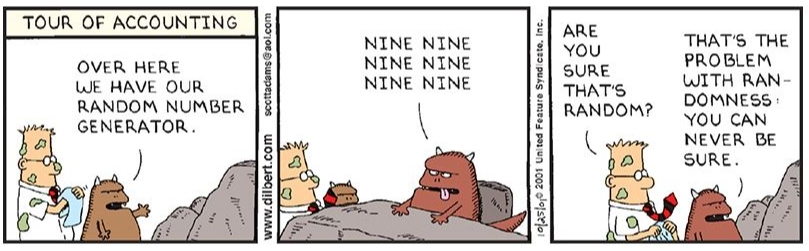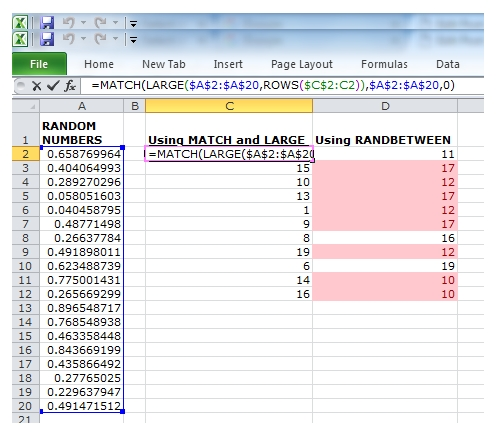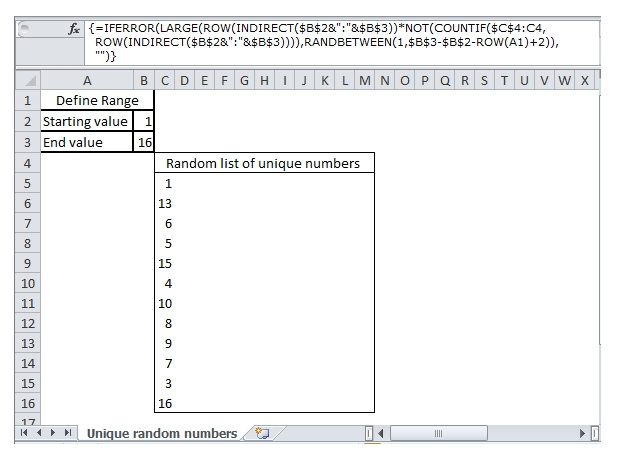#### Email Us

Follows

The human being cannot consciously generate random numbers sequences as they tend to avoid/favor picking specific numbers yet there is so much application of randomness in life.

Random numbers are useful in a number of ways, such as generating data encryption keys,GUIDS, Strong Passwords, selecting random samples or creating survey groups, generating lottery numbers e.t.c

Scientists, auditors, cryptographers, betting firms, polling firms, lottery players endeavor very hard to ensure the numbers they use are really random numbers as shown below by Scott Adams’s Dilbert cartoon.If you want to be sure the numbers you generate are random, use excel RAND and RANDBETWEEN functions.

►RAND function returns a random number between 0 and 0.99999999999999999. The function can generate up to 17 decimal places precision.

`Syntax = RAND()`

►RANDBETWEEN function returns values in a range you specify.

```Syntax = RANDBETWEEN(bottom, top)→→bottom(smallest integer) & top (largest integer)
```

### NOTES:

►RAND function can also be used to generate random numbers between zero and any k number.

`= RAND() * k+1`

For example to generate random numbers between 0 and 13,

`=RAND() * 13+1`

►RAND can also be used to generate random numbers in a given floor and a ceiling value just like RANDBETWEEN.

For example to generate random numbers between 34 and 64

`=RAND() * (64-34)+34`

►RANDBETWEEN is prone to generate duplicates, therefore if you want to generate unique numbers in given range use below;

• Create a helper row with random numbers use RAND() then use MATCH and LARGE to fetch the ROW numbers`=MATCH(LARGE(\$A\$2:\$A\$20,ROWS(\$C\$2:C12)),\$A\$2:\$A\$20,0)`

In this formula, MATCH is used to return the row which contains the Largest k number.

• If you do not want to use a helper row, then you can use array formula shown below
```{=IFERROR(
LARGE(
ROW(INDIRECT(\$J\$3&":"&\$J\$4))*
NOT(COUNTIF(\$F\$5:F5,ROW(INDIRECT(\$J\$3&":"&\$J\$4))))
,RANDBETWEEN(1,\$J\$4-\$J\$3-ROW(A1)+2)),"")}```